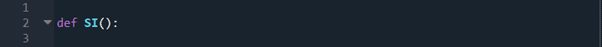## How to write python program to calculate simple interest

In this article we will learn How to write python program to calculate simple interest in step by step. Let’s see:

### Follow simple steps to write python program to calculate simple interest

Step 1 – Note down the formula of simple interest.
SI=(P×T×R)/100
Here, SI is simple interest
P is principal amount given for interest
T is time period for which P was given for interest
R is the rate of interest

Step 2 – Identify the variables in the formula.
We can clearly identify that P, T, R are the required variable.

Step 3 – Decide how the variables are going to get their values.
I am planning to give the variables their values by asking the user for input.

Step 4 – Decide how we will implement the above formula, namely through a class or a function or through simple sequential input, calculation of answer, and output.
I am going to define a function to calculate and return the value of the Simple interest calculated from the inputs given by user.

Step 5 – Decide the IDE we are going to use for programming.
I am going to use Spyder IDE which comes in the Anaconda distribution.

Step 6 –Declare the function, I am using SI as the function name.

#### How to write python program to calculate simple interest.Decide the IDE we are going to use for programming

Step 7 – Write statements to ask user for the values of P, T, R when the function is called.

Step 8 – Writing an “if name == ‘main’” block to call the function SI() in order to test it. “if name == ‘main‘” block runs the code in it only when the program is run directly and not imported.

Output:

Step 9 – We need to make sure that only numbers are inputted while asking for input.
To do that, I am going to put each input statement in its own while loop. The while loop will be exited when a number is inputted. Checking if the value inputted is correct is done by checking if it can be converted into a floating-point number. Since converting a string containing characters other than numbers into floating-point numbers results in a traceback, I am using try and except block to achieve this part.
In the try block I am trying to convert input into floating point value. If an error occurs then the flow jumps to except block where I am just going to ask the loop to continue. This goes on until a valid input is given.

Output :

Step 10 – We can further refine the code by printing the user to print valid inputs when he enters invalid inputs and telling the units in which we are taking inputs.

Output: